January 22, 2018

A Categorical Semantics for Causal Structure

Posted by John Baezguest post by Joseph Moeller and Dmitry Vagner

We begin the Applied Category Theory Seminar by discussing the paper A categorical semantics for causal structure by Aleks Kissinger and Sander Uijlen.

Compact closed categories have been used in categorical quantum mechanics to give a structure for talking about quantum processes. However, they prove insufficient to handle higher order processes, in other words, processes of processes. This paper offers a construction for a $\ast$-autonomous extension of a given compact closed category which allows one to reason about higher order processes in a non-trivial way.

We would like to thank Brendan Fong, Nina Otter, Joseph Hirsh and Tobias Heindel as well as the other participants for the discussions and feedback.

Preliminaries

We begin with a discussion about the types of categories which we will be working with, and the diagrammatic language we use to reason about these categories.

Diagrammatics

Recall the following diagrammatic language we use to reason about symmetric monoidal categories. Objects are represented by wires. Arrows can be graphically encoded as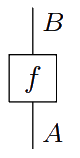Composition $\circ$ and $\otimes$ depicted vertically and horizontallysatisfying the properties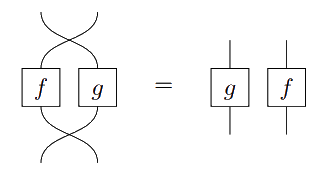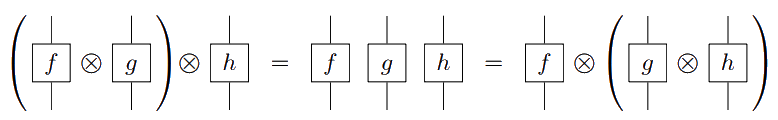and the interchange law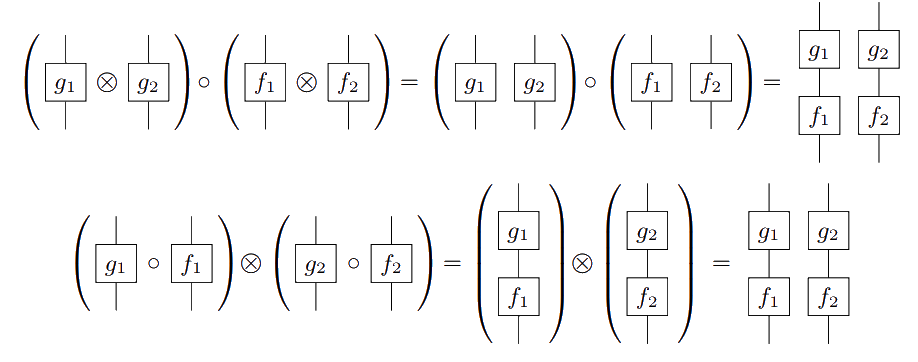If $I$ is the unit object, and $A$ is an object, we call arrows $I \to A$ states, $A \to I$ effects, and $I \to I$ numbers.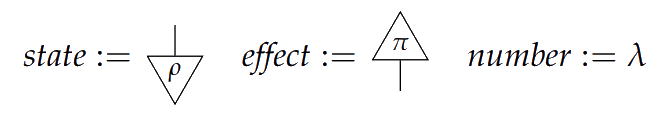The identity morphism on an object is only displayed as a wire, and both $I$ and its identity morphism are not displayed.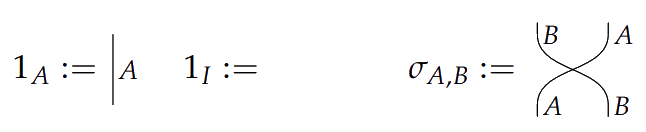Compact closed categories

A symmetric monoidal category is compact closed if each object $A$ has a dual object $A^\ast$ with arrows

$\eta_A \colon I \to A^\ast \otimes A,$

and

$\epsilon_A \colon A \otimes A^\ast \to I,$

depicted as $\cup$ and $\cap$ and obeying the zigzag identities: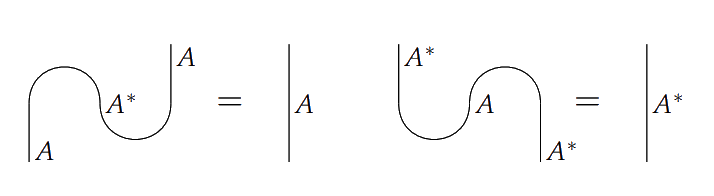Given a process $f \colon A \to B$ in a compact closed category, we can construct a state $\rho_f \colon I \to A^\ast \otimes B$ by defining

$\rho_f = (1_{A^\ast} \otimes f) \circ \eta_A.$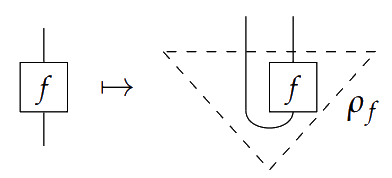This gives a correspondence which is called “process-state duality”.

An example

Let $Mat(\mathbb{R}_+)$ be the category in which objects are natural numbers, and morphisms $m \to n$ are $n\times m$ $\mathbb{R}_+$-matrices with composition given by the usual multiplication of matrices. This category is made symmetric monoidal with tensor defined by $n \otimes m = nm$ on objects and the Kronecker product of matrices on arrows, $(f \otimes g)^{kl}_{ij} = f^k_i g^l_j$. For example

$\begin{bmatrix} 1 & 2 \\ 3 & 4 \end{bmatrix} \otimes \begin{bmatrix} 0 & 5 \\ 6 & 7 \end{bmatrix} = \begin{bmatrix} 1\cdot \begin{bmatrix} 0 & 5 \\ 6 & 7 \end{bmatrix} & 2\cdot \begin{bmatrix} 0 & 5 \\ 6 & 7 \end{bmatrix} \\ 3 \cdot \begin{bmatrix} 0 & 5 \\ 6 & 7 \end{bmatrix} &4\cdot \begin{bmatrix} 0 & 5 \\ 6 & 7 \end{bmatrix} \end{bmatrix} = \begin{bmatrix} 0 & 5 & 0 & 10 \\ 6 & 7 & 12 & 14 \\ 0 & 15 & 0 & 20 \\ 18 & 21 & 24 & 28 \end{bmatrix}$

The unit with respect to this tensor is $1$. States in this category are column vectors, effects are row vectors, and numbers are $1\times 1$ matrices, in other words, numbers. Composing a state $\rho\colon 1 \to n$ with an effect $\pi\colon n \to 1$, is the dot product. To define a compact closed structure on this category, let $n^\ast := n$. Then $\eta_n \colon 1 \to n^2$ and $\varepsilon_n \colon n^2 \to 1$ are given by the Kronecker delta.

A Categorical Framework for Causality

Encoding causality

The main construction in this paper requires what is called a precausal category. In a precausal category, we demand that every system has a discard effect, which is a process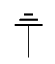$_A \colon A \to I$. This collection of effects must be compatible with $\otimes$:

•$_{A \otimes B} =$$_A$$_B$

•$_I = 1$

A process $\Phi \colon A \to B$ is called causal if discarding $B$ after having done $\Phi$ is the same as just discarding $A$.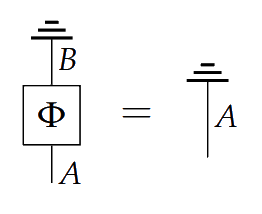If $A^\ast$ has discarding, we can produce a state for $A$ by spawning an $A$ and $A^\ast$ pair, then discarding the $A^\ast$: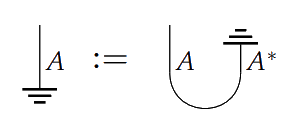In the case of $Mat(\mathbb{R}_+)$, the discard effect is given as row vector of $1$’s: $\mathbf{1}:=(1\cdots 1)$. Composing a matrix with the discard effect sums the entries of each column. So if a matrix is a causal process, then its column vectors have entries that sum to $1$. Thus causal processes in $Mat(\mathbb{R}_+)$ are stochastic maps.

A process $\Phi \colon A \otimes B \to A' \otimes B'$ is one-way signalling with $A \preceq B$ if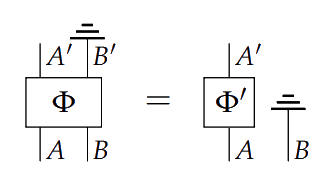and $B \preceq A$ if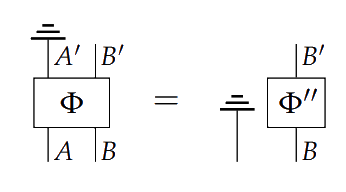and non-signalling if both $A\preceq B$ and $B\preceq A$.

The intuition here is that $A \preceq B$ means $B$ cannot signal to $A$; the formal condition encodes the fact that had $B$ influenced the transformation from $A$ to $A'$, then it couldn’t have been discarded prior to it occurring.

Consider the following example: let $A$ be a cup of tea, and $B$ a glass of milk. Let $\Phi$ the process of pouring half of $B$ into $A$ then mixing, to form $A'$ milktea and $B'$ half-glass of milk. Clearly this process would not be the same as if we start by discarding the milk. Our intuition is that the milk “signalled” to, or influenced, the tea, and hence intuitively we do not have $A \preceq B$.

A compact closed category $\C$ is precausal if

1) Every system $A$ has a discard process$_A$

2) For every system $A$, the dimension is invertible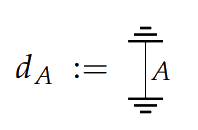3) $\C$ has enough causal states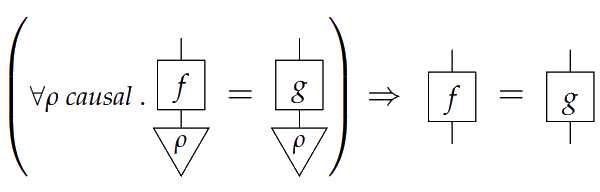4) Second order causal processes factor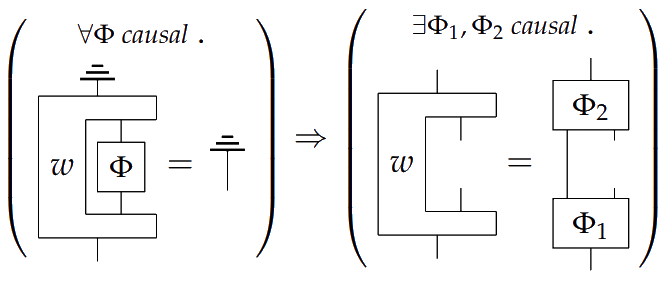From the definition, we can begin to exclude certain causal situations from systems in precausal categories. In Theorem 3.12, we see that precausal categories do not admit ‘time-travel’.

Theorem   If there are systems $A$, $B$, $C$ such that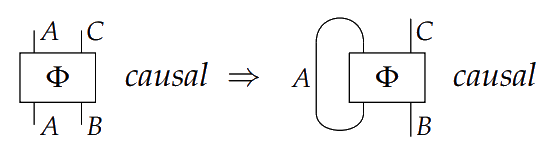then $A \cong I$.

In precausal categories, we have processes that we call first order causal. However, higher order processes collapse into first order processes, because precausal categories are compact closed. For example, letting $A \Rightarrow B := A^\ast\otimes B$,

$(A\Rightarrow B)\Rightarrow C = (A^\ast\otimes B)\ast\otimes C \cong B\Rightarrow A\otimes C$

We can see this happens because of the condition $A \otimes B \cong (A^\ast \otimes B^\ast)^\ast$. Weakening this condition of compact closed categories yields $\ast$-autonomous categories. From a precausal category $\C$, we construct a category $Caus[\C]$ of higher order causal relations.

The category of higher order causal processes

Given a set of states $c \subseteq \C(I,A)$ for a system $A$, define its dual by

$c^\ast = \{\pi \in A^\ast |\, \forall \rho \in c ,\, \pi \circ \rho = 1\} \subseteq \C(I, A^\ast)$

Then we say a set of states $c \subseteq \C(I,A)$ is closed if $c=c^{\ast\ast}$, and flat if there are invertible scalars $\lambda$, $\mu$ such that $\lambda$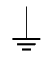$\in c$, $\mu$$\in c^\ast$.

Now we can define the category $Caus[\C]$. Let the objects be pairs $(A, c_A)$ where $c_A$ is a closed and flat set of states of the system $A \in \C$. A morphism $f \colon (A, c_A) \to (B, c_B)$ is a morphism $f \colon A \to B$ in $\C$ such that if $\rho \in c_A$, then $f \circ \rho \in c_B$. This category is a symmetric monoidal category with $(A, c_A) \otimes (B, c_B) = (A \otimes B, c_{A \otimes B})$. Further, it’s $\ast$-autonomous, so higher order processes won’t necessarily collapse into first order.

A first order system in $Caus[\C]$ is one of the form $(A, \{$$_A\}^\ast)$. First order systems are closed under $\otimes$. In fact, $C_C$ admits a full faithful monoidal embedding into $Caus[\C]$ by assigning systems to their corresponding first order systems $A \mapsto (A, \{$$_A\})$.

For an example of a higher order process in $Caus[Mat(\mathbb{R}_+)]$, consider a classical switch. Let

$\rho_0 = \begin{bmatrix} 1\\0 \end{bmatrix}, \quad \rho_1 = \begin{bmatrix} 0\\1 \end{bmatrix}, \quad \rho'_i = \rho_i^T,$

and let $s$ be the second order process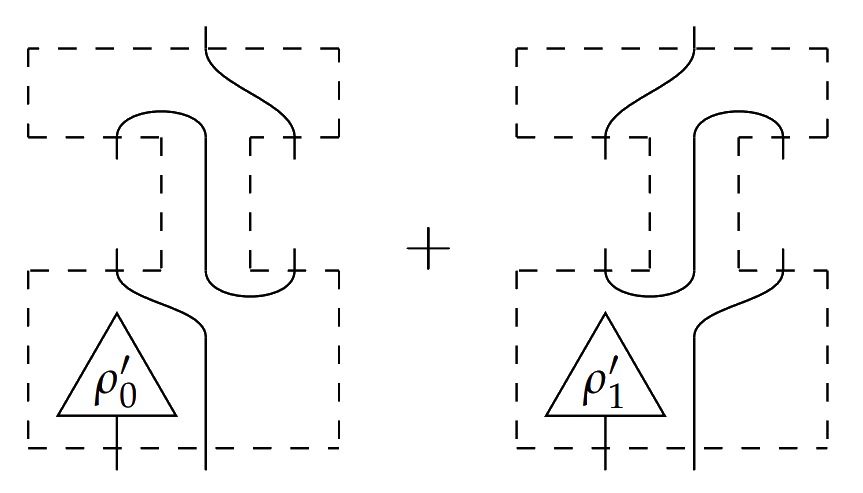This process is of type $X \otimes C \multimap (A \multimap B) \otimes (B \multimap A) \multimap C'$, where the two middle inputs take types $A \multimap B$ on the left and $B \multimap A$ on the right. Since $\rho'_i \circ \rho_j$ is $1$ if $i=j$ and $0$ otherwise, then plugging in either $\rho_0$ or $\rho_1$ to the bottom left input switches the order that the $A \multimap B$ and $B \multimap A$ processes are composed in the final output process. This second order process is causal because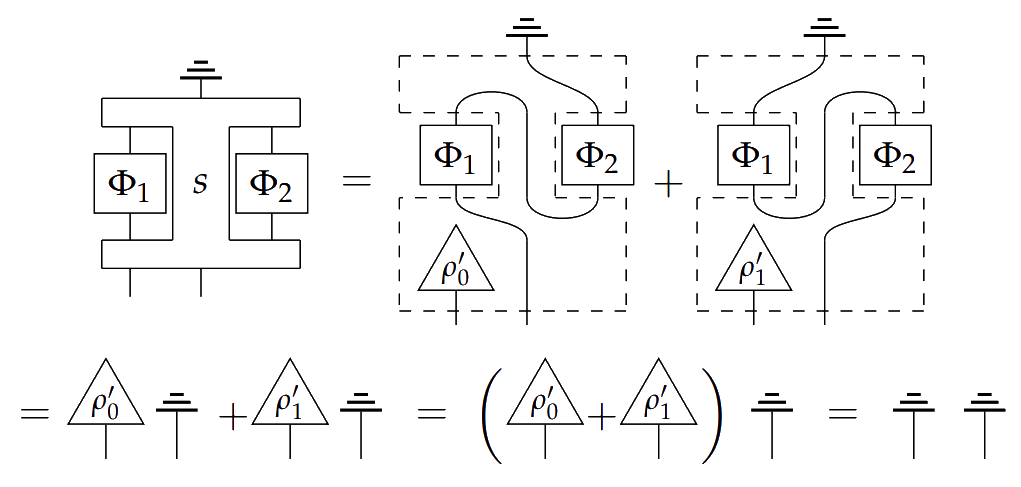The authors go on to prove in Theorem 6.17 that a switch cannot be causally ordered, indicating that this process is genuinely second order.

Posted at January 22, 2018 9:26 PM UTC

TrackBack URL for this Entry:   https://golem.ph.utexas.edu/cgi-bin/MT-3.0/dxy-tb.fcgi/3008

Re: A Categorical Semantics for Causal Structure

This was a very enjoyable read!

As you write, a process is causal if when we do it and then chuck the result, its the same as having chucked the input. I can see this as saying that the result of the process is really everything the process effects; getting rid of the result is the same as not having done the process at all. But I’m struggling to see how this relates to causality – probably because I don’t have much of an intuition for what a “causal process” is pre-theoretically. Would ya’ll be willing to elaborate on how this definition of a causal process deserves the name?

Posted by: David Jaz Myers on January 22, 2018 11:35 PM | Permalink | Reply to this

Re: A Categorical Semantics for Causal Structure

At first I thought “effects” here was a misspelling for “affects”, but this seems to be one of the rare cases where either verb is potentially suitable, even though they mean different things!

Posted by: John Baez on January 23, 2018 12:35 AM | Permalink | Reply to this

Re: A Categorical Semantics for Causal Structure

Posted by: Tom Leinster on January 23, 2018 12:47 AM | Permalink | Reply to this

Re: A Categorical Semantics for Causal Structure

Posted by: test on January 23, 2018 9:41 AM | Permalink | Reply to this

Re: A Categorical Semantics for Causal Structure

Posted by: John Baez on January 23, 2018 6:54 AM | Permalink | Reply to this

Re: A Categorical Semantics for Causal Structure

Maybe we could say that a causal process is a process that effects everything it affects :)

Posted by: David Jaz Myers on January 25, 2018 4:27 PM | Permalink | Reply to this

Re: A Categorical Semantics for Causal Structure

But I’m struggling to see how this relates to causality – probably because I don’t have much of an intuition for what a “causal process” is pre-theoretically. Would ya’ll be willing to elaborate on how this definition of a causal process deserves the name?

A way I’ve seen it explained is this: what if a process is not causal? Then discarding the result of the process is not the same simply discarding, meaning the process could be affecting something that isn’t encoded in its outputs. So a causal process is one where all systems it affects are encoded in the output.

Posted by: Joe Moeller on January 23, 2018 7:05 AM | Permalink | Reply to this

Re: A Categorical Semantics for Causal Structure

In programming parlance, a causal process is “purely functional”.

Posted by: Mike Stay on January 25, 2018 12:29 AM | Permalink | Reply to this

Re: A Categorical Semantics for Causal Structure

A way I’ve seen it explained is this: what if a process is not causal? Then discarding the result of the process is not the same simply discarding, meaning the process could be affecting something that isn’t encoded in its outputs. So a causal process is one where all systems it affects are encoded in the output.

So, in particular, if I think of the output as happening “after” the process (is done), then a causal process is one all of whose effects occur after it.

Posted by: David Jaz Myers on January 25, 2018 4:23 PM | Permalink | Reply to this

Re: A Categorical Semantics for Causal Structure

In physics there’s been an extensive study of causality, especially in the context of special relativity — particularly in the study of relativistic quantum field theory, where there are some axiom systems that include axioms governing causality. For example, the Haag–Kastler axioms include an axiom of causal locality. I wonder if Kissinger’s approach has been related to this work in physics. It would be great if models of the Haag–Kastler axioms gave examples of categories obeying some of Kissinger’s properties.

Posted by: John Baez on January 23, 2018 6:53 AM | Permalink | Reply to this

Re: A Categorical Semantics for Causal Structure

Posted by: David Corfield on January 23, 2018 9:06 AM | Permalink | Reply to this

Re: A Categorical Semantics for Causal Structure

Typos:

The numbering of conditions in the definition of a compact closed category being precausal is 1, 2, 1, 1.

Then in “Given a set of states $c \subseteq \C(I,C)$ for a system $A$”, the $C$ should be $A$.

Posted by: David Corfield on January 23, 2018 8:58 AM | Permalink | Reply to this

Re: A Categorical Semantics for Causal Structure

Posted by: Joe Moeller on January 23, 2018 11:32 PM | Permalink | Reply to this

Re: A Categorical Semantics for Causal Structure

Posted by: John Baez on January 24, 2018 6:10 AM | Permalink | Reply to this

Re: A Categorical Semantics for Causal Structure

This is really interesting and I would like to understand it, especially because I’ve recently been thinking a lot about $\ast$-autonomous categories. But I am confused about what a “higher-order process” is. Did you define it? Do I have to read the paper to find out what it is?

Posted by: Mike Shulman on January 26, 2018 4:58 PM | Permalink | Reply to this

Re: A Categorical Semantics for Causal Structure

I too am confused about this first-order vs. second-order distinction. If this is important, there should be some stuff one can say that would help explain the idea in rough terms. The blog article gives a formal definition of ‘first-order’ (which I don’t really grok), but doesn’t define the concept of ‘second-order’.

Posted by: John Baez on January 26, 2018 9:12 PM | Permalink | Reply to this

Re: A Categorical Semantics for Causal Structure

At first, when we’re just in a precausal category, $C$, you think of a first order process as just a morphism in the category, and a higher order process like a map from a set of processes to a set of processes, or from a set of processes to an object (I think). You can convince yourself this is possible by manipulating the string diagrams. The problem is that they prove that all higher order structure collapses to first order structure as long as you’re working in a compact closed category.

This is really the reason $Caus[C]$ is constructed. The compact closed category $C$ embeds monoidally into $Caus[C]$, but $Caus[C]$ is $\ast$-autonomous, so higher order things at least stand a chance. So then we call things that are in $C$ (or really, things hit by the embedding) first order, and other things are higher order. This is how I understood it at least.

Posted by: Joe Moeller on January 27, 2018 1:17 AM | Permalink | Reply to this

Re: A Categorical Semantics for Causal Structure

Posted by: Mike Shulman on January 27, 2018 10:53 PM | Permalink | Reply to this

Re: A Categorical Semantics for Causal Structure

Joseph and Dmitri should answer that question, but I broke down and peeked at the paper, and found it somewhat cryptic concerning this concept of “higher-order process”. Here are the first two instances of the term “higher-order” in this paper.

First:

The key ingredient in studying (and varying) causal structures of processes is the development of a coherent theory of _higher order causal processes, i.e. maps from causal processes to causal processes. For example, if we treat local agents (or events, laboratories, etc.) as first order causal processes, then the act of composing these processes in a particular causal order should be treated as a second-order process. In this paper, we develop a categorical framework for expressing and reasoning about such higher order processes.

and then:

We will begin with a category C and construct a new category Caus[C] of higher-order causal processes, into which the category of (first-order) causal processes satisfying the equation above embeds fully and faithfully.

So, if you’re willing to buy this category Caus[C], then a “higher-order causal process” is defined as a morphism in there.

Posted by: John Baez on January 27, 2018 11:09 PM | Permalink | Reply to this

Re: A Categorical Semantics for Causal Structure

But (again I’m reading only the blog post) $Caus[C]$ is defined assuming $C$ is “precausal”, and the definition of “precausal” already refers to “second-order processes”. So it seems circular to define a higher-order process as a morphism in $Caus[C]$.

Posted by: Mike Shulman on January 28, 2018 6:00 AM | Permalink | Reply to this

Re: A Categorical Semantics for Causal Structure

Hi, My understanding is as follows. A compact closed category does admit higher types, but because of compact closedness they are isomorphic to first order types.

So in a compact closed category, there are second order morphisms, such as $w:(A\multimap B)\to (C\multimap D)$ in the definition of “precausal”, although they could also be thought of as first order $w:A^* \otimes B\to C^* \otimes D$. The diagrammatic notation is explained on page 4 of the paper. On the other hand the “causal” category is *-autonomous but not compact closed, and so higher order is genuinely different from first order there.

Incidentally, in case it helps, and as it also says in the paper: this is a variation of the double gluing construction. I think it’s really nice to see a new and compelling application of this proof theoretic idea.

Posted by: Sam S on January 28, 2018 9:29 AM | Permalink | Reply to this

Re: A Categorical Semantics for Causal Structure

This makes it sound like the notion of “higher-order” process is not a semantic one but only a syntactic one. If a first-order process is just a morphism in the category, and a higher-order one is a morphism whose domain and codomain involve $\multimap$, then semantically a higher-order process would be a special case of a first-order one, which doesn’t seem right. The only way to distinguish morphisms “whose domain and codomain don’t involve $\multimap$” to be the “first-order” ones is syntactic. Is that right?

Also, in a $\ast$-autonomous category we do have $(A\multimap B) = (A^\ast \invamp B) = (A \otimes B^\ast)^\ast$, so I don’t see why it would be any different than a compact-closed one in terms of “reducing higher-order to first-order”.

Posted by: Mike Shulman on January 28, 2018 6:21 PM | Permalink | Reply to this

Re: A Categorical Semantics for Causal Structure

Just to pick up on the last comment, about star-autonomous versus compact closed. Usually the “order” of an intuitionistic proposition is defined by something like $o(A\to B)=max(o(A)+1,B)$, as I guess you know. In MLL you can have interesting nestings of $\otimes$ and $\parr$, even in negation normal form. So you can count the max alternations in any branch of a formula, and we could call this the order of an MLL formula. And then in a compact closed situation every formula is isomorphic to a first order one, but not so in an arbitrary *-autonomous situation. Unless I am really missing the point!

Perhaps “order” in this sense is a syntactic thing, and not up-to a naive isomorphism. It is saying something about the complexity of an interaction protocol with which the object should be considered. I would be very interested to see a more semantic or universal characterization.

Posted by: Sam S on January 29, 2018 11:34 AM | Permalink | Reply to this

Re: A Categorical Semantics for Causal Structure

Here’s another way to ask the question: what is the complete and precise definition of “precausal category”? The definition in the blog post seems complete except that axiom (4) involves the notion of a “second order process”, which was not defined.

Posted by: Mike Shulman on January 28, 2018 6:23 PM | Permalink | Reply to this

Re: A Categorical Semantics for Causal Structure

The way I understand this construction and the notion of higher-order process is as follows: you start with a compact closed category which is a sort of wild-west where information flow between processes in unconstrained in the sense that there is no privileged direction and total symmetry between inputs and outputs. In this context, there are no notions of higher-order processes because every type reduces to a monoidal product of basic types.

But you have a distinguished discarding process that you use to test the direction of information flow. The causality axiom introduces a direction and the possibility of talking about higher-order processes in a consistent way, by breaking the symmetry between inputs and outputs. I think this is what the authors anticipate (without defining it clearly first) when then use the phrase “second-order causal processes factorise” in the (C4) axiom: the definition of a second-order process is implicit in the left-side of the implication: it is a process $w: A \otimes D \rightarrow C\otimes B$ or, equivalently $w: A\otimes (D\otimes C^*) \rightarrow B$ —where, in the picture, $A$ and $B$ are the bottom and top wire, and $D$ and $C$ are the internal wires in the hole, understood as the domain and codomain of the first-order process it takes as input—such that, if we discard its output $B$ on a causal input process $\Phi: C \rightarrow D$, it is the same as having done nothing. This feels like a genuine second-order definition which is not just syntactic. You can extend it to higher-order and this is precisely what the paper’s construction does.

It is close in spirit to existing semantics of linear logic which are constructed by getting rid of certain cycles in the syntax (one can find similar acyclicity conditions for the correctness of proof nets or in the nilpotency condition of the geometry of interaction). Intuitively, it makes sense since cycles prevent us from interpreting domain and codomain as genuine inputs and outputs. I like this approach because we don’t need to make assumptions about causality: it becomes a property of some processes. This has also been a fruitful approach in other fields that have been discussed here, such as control theory.

Posted by: Robin Piedeleu on January 29, 2018 9:24 AM | Permalink | Reply to this

Re: A Categorical Semantics for Causal Structure

The left half of the picture that follows axiom 4 is supposed to define second order causal process.

Posted by: Joe Moeller on January 29, 2018 7:49 PM | Permalink | Reply to this

Re: A Categorical Semantics for Causal Structure

Thanks for your help everyone. I think I mostly understand it now, and (in the compact closed case) it is what I would call a “syntactic” notion. If you have a morphism $f:X\to Y$, then you can call it first-order; but if I then tell you that $X = (A\multimap B)$ and $Y = (C\multimap D)$, then you realize that in fact (relative to $A,B,C,D$) we can say that $f$ is higher-order. So the “order” is not a property of $f$ itself, but of how we choose to decompose its domain and codomain using $\otimes,\multimap$, and I call that syntactic. In particular, by forgetting any such decomposition, we can regard anything as being first-order.

Also, this is really not a property of a morphism, but of an object: $X$ is first-order, $A\multimap B$ is second-order. In other words, the order is not really a property of a process, but of the type of a process; though of course since every process has a type, it also has an order.

In a general $\ast$-autonomous category, I believe that order will still be syntactic, and we can still regard anything as first-order by forgetting any syntactic decomposition. However, it seems that in the particular $\ast$-autonomous category $Caus[C]$, we can make the order into a semantic notion by redefining it. In $Caus[C]$ we have a subcategory (equivalent to $C$) that we can define to be the “first-order objects”, and then the objects outside this subcategory (particularly those obtained by applying $\multimap$ to first-order objects) are “higher-order”.

I think the reason $\ast$-autonomy versus compact closed is important is that in a compact closed category, if we try to identify a subcategory of “first order objects” that are closed under $\otimes$, then they will automatically also be closed under $\multimap$ too (at least, modulo rearranging domains and codomains), so that we can’t get to any new (i.e. non-first-order) “higher-order” objects by applying $\multimap$. I think this is what the blog post means by “higher order processes collapse into first order processes”. In a $\ast$-autonomous category, we can have a subcategory of “first order objects” that’s closed under $\otimes$, but not under $\parr$ and hence not under $\multimap$, so that we can get non-first-order processes by applying $\multimap$ to first-order ones.

Posted by: Mike Shulman on January 30, 2018 12:35 PM | Permalink | Reply to this

Re: A Categorical Semantics for Causal Structure

Hi all,

Great summary Joe & Dmitry, and great discussions. I would chime in to clarify some points, but it looks like you all have worked it all out already. :)

I’ll just sum things up a bit and make a few remarks:

HIGHER-ORDER PROCESSES

In the context of this paper, we use the term “higher order process” to refer to any morphism in Caus[C]. This includes:

1. first order processes, which are morphisms whose domain and codomain are first-order systems (i.e. those of the form (A, {discard}*) )

2. second order processes: morphisms whose domain and codomain are of the form (A -o B) for A and B first-order systems.

….and lots of other stuff. This is not a purely syntactic notion, because it relies on a fixed, concrete notion of what first-order means. It is useful to think of first-order systems as a sort of “atomic” type in the logic, which you can build more complicated things out of.

At some point, we were going back and forth between defining the objects of Caus[C] to be pairs (A,c) where c is flat and closed, or instead letting them be generated inductively from first order objects (A, {discard}*) using tensor and star. Since all such objects are pairs (A,c) where c is flat and closed, this would have yielded a smaller category than Caus[C] we defined (whose morphisms maybe had more right to call themselves “higher-order processes”). In the end, we opted for the non-inductive definition for the sake of simplicity, at the cost of Caus[C] possibly containing some “weird” systems (A,c) which don’t arise inductively from first-order ones.

Mike is right that there is a certain circularity to the definition of Caus[C], because precausal categories require that second-order causal processes factorise (where second-order causal processes are a Caus[C] concept). This chicken v. egg situation is solved by the fact that second-order causal is indeed defined by the LHS of the implication, as Robin points out. Put another way, the words in axioms (C3) and (C4) are only meant to give intuition behind these axioms. The formal content is precisely contained in the formulas.

CAUSALITY

Probably the most controversial aspect of this line of work is our insistence on calling the “discard output = discard input” equation the causality postulate. We do this for a couple of reasons.

The terminology originates in operational probabilistic theories, where (an equivalent version of) this equation is given the following interpretation, in terms of an abstract notion of measurement: “Causality: the probability of a measurement outcome at a certain time does not depend on the choice of measurements that will be performed later.” (see arXiv:1011.6451)

There is indeed a connection to special relativity. Namely, if you form a directed acyclic diagram of processes satisfying the causality postulate, then the overall process will respect the evident “non-signalling” constraints between certain inputs and outputs given by this diagram. Coecke, Hoban, and I explained this in some detail in arXiv:1708.04118. There, we call the causality postulate “process terminality” to avoid overloading the term “causality” too much.

So, toward addressing John’s question about the relationship to Haag-Kastler axioms: this obeying of non-signalling constraints, which is equivalent to our causality equation, seems to be most closely related to the Haag-Kastler axiom of causal locality, which states that spacelike subalgebras commute. For quantum-like C*-algebras, this is the same as non-signalling, as established by Clifton, Bub, and Halverson (arXiv:0211089).

Posted by: Aleks Kissinger on January 31, 2018 1:50 PM | Permalink | Reply to this

Re: A Categorical Semantics for Causal Structure

Thanks for that explanation, Aleks! Now that I think I know what the definition of $Caus[\mathbf{C}]$ is saying, I want to understand it. To that end, let me try to dissect it using the sterile scalpel of pure category theory. Specifically, I will try to reinvent this definition wearing the hat of a category theorist who doesn’t know anything about “causality” or “processes”.

There is a straightforward way to try to make any closed symmetric monoidal category $\mathbf{D}$ into a $\ast$-autonomous one. Namely, pick an object $\bot$ and consider the subcategory of objects $X\in \mathbf{D}$ for which the double-dualization map $X \to (X\multimap\bot)\multimap \bot$ is an isomorphism. I don’t know the most general conditions under which this works, but one general case in which I’m pretty sure it works is when the self-duality adjunction $(-\multimap\bot)\dashv (-\multimap\bot)$ is idempotent, since then the subcategory in question is closed under $\multimap$, reflective (hence monoidal), and contains $\bot$. (An even more particular case of this is when $\mathbf{D}$ is a poset, since then all adjunctions are idempotent. This includes Girard’s original phase semantics of linear logic.)

Of course if $\mathbf{D}$ is already $\ast$-autonomous, with $\bot$ its dualizing object, then this construction gives $\mathbf{D}$ back again, and in particular that happens if $\mathbf{D}$ is compact closed (with $\bot = I$). So if we want to make a new $\ast$-autonomous category out of a compact closed one, we have to combine this construction with something else, and a natural choice is some sort of “free” or “universal” construction on our compact closed category $\mathbf{C}$. The first thing that would come to mind for me is the Yoneda embedding (with Day convolution), but another important such “universal” construction is Artin gluing and more specifically the scone. In the non-cartesian monoidal case, this means the comma category $scn(\mathbf{C}) = (Set\downarrow \mathbf{C}(I,-))$, whose objects are triples $(A,X,X\to \mathbf{C}(I,A))$.

If $A$ is closed symmetric monoidal, so is $scn(\mathbf{C})$. (More generally, if $F$ is colax monoidal and $G$ is lax monoidal, then the comma category $(F\downarrow G)$ is monoidal.) The tensor product is straightforward:

$(A,X, X\to \mathbf{C}(I,A)) \otimes (B,Y, Y\to \mathbf{C}(I,B)) = (A\otimes B, X\times Y, X\times Y \to \mathbf{C}(I,A) \times \mathbf{C}(I,B) \to \mathbf{C}(I,A\otimes B).$

The internal-hom $(A,X, X\to \mathbf{C}(I,A)) \multimap (B,Y, Y\to \mathbf{C}(I,B))$ is $A\multimap B$ equipped with the map $Z \to \mathbf{C}(I,A\multimap B) \cong \mathbf{C}(A,B)$ whose fiber over $f:A\to B$ is the set of liftings of $f$ to a morphism in $scn(\mathbf{C})$, i.e. the set of maps $X\to Y$ making the evident square commute.

Thus, from any closed symmetric monoidal category $\mathbf{C}$, if we pick an object $\bot$ and a function $Z\to \mathbf{C}(I,\bot)$, giving an object $\bot'$ of $scn(\mathbf{C})$, and we can then ask whether the induced adjunction $(-\multimap\bot')\dashv (-\multimap\bot')$ on $scn(\mathbf{C})$ is idempotent. Since $\multimap$ on $scn(\mathbf{C})$ acts as the original $\multimap$ on the $\mathbf{C}$ components, this requires the original adjunction $(-\multimap\bot)\dashv (-\multimap\bot)$ on $\mathbf{C}$ to also be idempotent. And since the fixed points of $(-\multimap\bot')$ will have fixed points of $(-\multimap\bot)$ as their $\mathbf{C}$ components, we might as well restrict ourselves to that latter category of fixed points to start with, which is to say we might as well assume that $\mathbf{C}$ is itself $\ast$-autonomous with $\bot$ its dualizing object.

This ensures that the double $\bot'$-dualization map is always an isomorphism on $\mathbf{C}$ components. To ensure that this adjunction is idempotent, therefore, it remains to consider the set components, and one natural way to make this happen is to make that “part” of the adjunction posetal. It certainly works if we simply restrict to the subcategory of $scn(\mathbf{C})$ where the function $X\to \mathbf{C}(I,A)$ is injective. I think it should also work if we assume merely that the map $Z\to \mathbf{C}(I,\bot)$ is injective, since then any hom-object $(A,X,c_A) \multimap \bot'$ will also have this property; but then any fixed point of $(-\multimap \bot')$ will also have this property, so we might as well have restricted to that subcategory in the first place.

So now what we have is a construction of a new “$\ast$-autonomous scone” starting from any $\ast$-autonomous category $\mathbf{C}$ equipped with a subset $Z$ of $\mathbf{C}(I,\bot)$. Its objects are objects $A\in \mathbf{C}$ equipped with a subset $c \subseteq \mathbf{C}(I,A)$ such that $c = c^{\ast\ast}$, where $c^{\ast}\subseteq \mathbf{C}(I,A^\ast) \cong \mathbf{C}(A,\bot)$ is the set of maps $\pi:A\to \bot$ such that for all $(\rho : I \to A) \in c$, the composite $I \xrightarrow{\rho} A \xrightarrow{\pi} \bot$ lies in $Z$. In particular, this applies when $\mathbf{C}$ is compact closed, with $\bot=I$, and where we can take $Z$ to be the singleton containing the identity, reproducing the main part of the definition of $Caus[\mathbf{C}]$.

So far we haven’t used any “discard processes” or any of the axioms of precausal-ness, and the only thing that’s missing from the definition of $Caus[\mathbf{C}]$ is the “flatness” condition (which refers to the discard processes). So that leads me to ask questions like:

1. Why are the “flat” objects closed under the $\ast$-autonomous structure of the scone? Presumably this uses the axioms of precausal-ness; does it use all of them?

2. What purpose does the “flatness” restriction serve?

3. For any scone there is a projection $scn(\mathbf{C}) \to \mathbf{C}$ preserving all the structure, and it’s interesting to ask to what extent (if any) it has a section. The blog post mentions that $C_C$ (what is that? The subcategory of causal maps?) embeds fully-fathfully in $Caus[\mathbf{C}]$; presumably this is a (partial?) section of this projection. How much of the axioms of precausal-ness does this statement use? Does it use all of them? If we assume only that such a (partial?) section exists, how much of the axioms of precausal-ness can we recover?

Posted by: Mike Shulman on January 31, 2018 10:42 PM | Permalink | Reply to this

Re: A Categorical Semantics for Causal Structure

Just to connect terminology: I think what you call “new *-autonomous scone” is elsewhere called “tight orthogonality subcategory of the glued category” for a “focussed orthogonality” (e.g. in Hyland & Schalk, Glueing and Orthogonality for Models of Linear Logic, p32).

Posted by: Sam S on February 1, 2018 4:28 PM | Permalink | Reply to this

Re: A Categorical Semantics for Causal Structure

I’ll see if I can answer some of these questions, and try not to get cut. :)

1. Flatness is a pretty robust concept. All it depends on is that fact that the “raw materials” category C is compact closed, and precausal axiom (C1), namely that C is equipped with discarding maps $d_A : A \to I$, and they respect the monoidal structure. Then, preservation under tensor is immediate, and preservation under (-)* follows from the fact that the definition of flat is symmetric under taking the (compact closed) star of everything.

2. This is a bit more mysterious. Any object built up inductively from first-order objects and the *-autonomous structure is flat, however the converse is not true. So, flatness might not be the One True Definition needed for the Caus[C] construction.

Intuitively, it is saying something like both c and c* have something that plays the role of a “top” element (or a “bottom” element, depending on how you order things). In the concrete model of stochastic maps, that element for first-order systems is the uniform distribution, for systems A -o B, this is the constant function on to the uniform distribution (aka the perfectly noisy channel) and so on.

In particular, it guarantees that for any system (X, c), there is a canonical map in Caus[C] from (X,c) into the associated first-order system (X, {$d_X$}*) which is given by scalar multiplication (i.e. “renormalising”).

A recurring theme in the paper is to start with a map between higher-order systems, use flatness to reduce the problem to first-order systems, then apply precausal axiom (4) to produce a factorisation.

A big part of the story of causality is about factorisation. If a process factors in a certain way, this might not necessarily say certain causal relationships exist, but it does rule out certain possibilities, since there might be e.g. no way for information to flow from a certain input to a certain output. If you can rule out enough possibilities, you might be able to say something meaningful about the possibilities that are left. In statistical inference, this is basically the heart of the “constraint-based approach” to discovering causal relationships from data.

But if you are using your scalpel correctly, I shouldn’t be telling you this. :)

3. Indeed, $C_c$ is the (wide) subcategory of C consisting just of those morphisms which satisfying the “causality postulate”, namely $d_B \circ f = d_A$. This gives a “partial” section to the projection of Caus[C] down to C (which I tend to think of as a forgetful functor, since it forgets the constraint c from the pair (X,c)).

I think this section will already exist if you just have precausal axioms (C1) and (C3). Hence, its probably pretty easy to cook up examples which do have such a section, but are not precausal. For example, the category Rel of sets and relations (with tensor = cartesian product) only satisfies a weaker form of axiom (C4), called (C4’) in the paper, but still looks like a very interesting example to work with.

Annoyingly, flatness breaks the “natural” choice for giving the forgetful functor left and right adjoints, namely sending A to (A, {}) and (A, C(I,A)), respectively. Neither of these sets are flat in general.

Posted by: Aleks Kissinger on February 1, 2018 2:13 PM | Permalink | Reply to this

Re: A Categorical Semantics for Causal Structure

I didn’t mean to give the impression that my scalpel-equipped category theorist hat shouldn’t pay any attention to the applications. On the contrary: I think category theory is at its best when it starts from what people actually see in applications and then finds the best abstract way to describe it, rather than trying to force things unnaturally into some already-existing abstract context. But I also think it’s an interesting exercise to try to develop as much as possible of a construction without reference to applications, in particular to find out how “unavoidable” it is (and how much more generally applicable).

It sounds as though in this case, that exercise will stop before the imposition of flatness. There’s nothing wrong with that, although it suggests to me, as you say, that flatness may not be the One True Definition. For instance, it sounds as though if you leave out flatness you get a category with nicer abstract properties (e.g. the existence of certain adjoints — and although I haven’t thought about this, it might be more likely to have limits and colimits), and as John Baez famously said (attributing the idea to Grothendieck), “it’s better to work with a nice category containing some nasty objects, than a nasty category containing only nice objects”. I’m looking forward to reading the paper that Sam mentioned.

Posted by: Mike Shulman on February 1, 2018 6:08 PM | Permalink | Reply to this

Re: A Categorical Semantics for Causal Structure

Thanks for attributing that to me! I wonder if Grothendieck ever said something like that, or whether he just behaved as if he believed it, for example when inventing the category of schemes (to contain the varieties as specially nice objects) and the topos of set-valued sheaves (to contain sheaves of abelian groups, etc. as specially nice objects).

Posted by: John Baez on February 1, 2018 7:10 PM | Permalink | Reply to this

Re: A Categorical Semantics for Causal Structure

In some form that philosophy goes back way before Grothendieck. It’s been absolutely fundamental to analysis since the early 20th century — e.g. working in the Banach space completion of a normed space of functions, even if it’s not clear how to interpret the points in that completion as functions themselves.

Although I believe Grothendieck himself sometimes spoke as though his work in the algebraic world came after a clean break with his earlier work in functional analysis, I wonder how much his later mathematics actually owed to that background.

Posted by: Mark Meckes on February 1, 2018 9:46 PM | Permalink | Reply to this

Re: A Categorical Semantics for Causal Structure

The nLab records a quotation attributed to Deligne:

If the decision to let every commutative ring define a scheme gives standing to bizarre schemes, allowing it gives a category of schemes with nice properties.

(From Quelques idées maîtresses de l’œuvre de A. Grothendieck_, Matériaux pour l’histoire des mathématiques au XX$^e$ siecle (Nice, 1996), Societé Mathématique de France, 1998, pp. 11–19)

Not quite as pointedly expressed as the quote attributed to Baez, but it seems likely that this is close to how Grothendieck felt.

Posted by: Todd Trimble on February 2, 2018 11:51 AM | Permalink | Reply to this

Post a New Comment